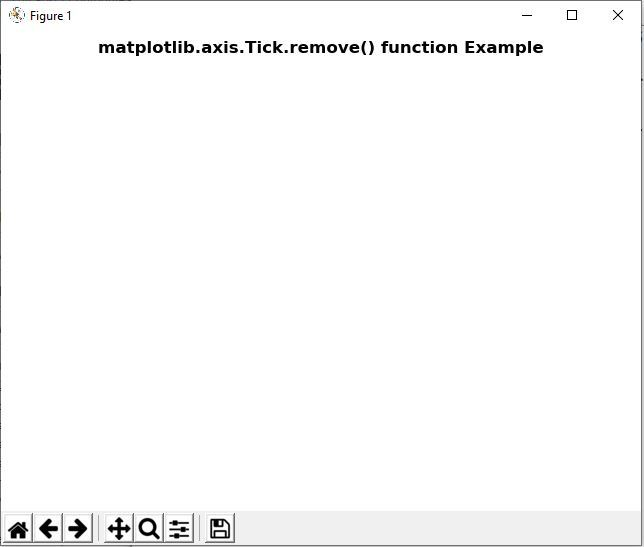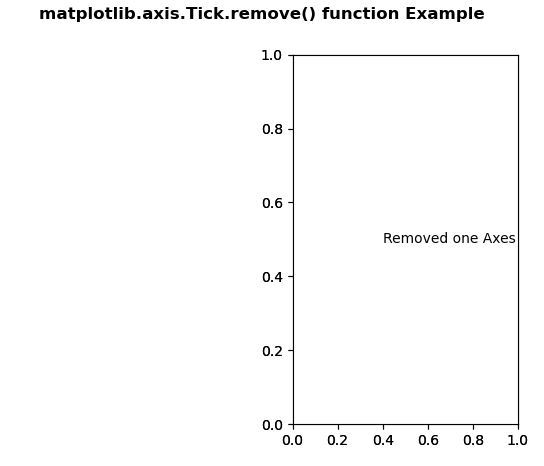Related Articles

# Matplotlib.axis.Tick.remove() function in Python

• Last Updated : 10 Jun, 2020

Matplotlib is a library in Python and it is numerical – mathematical extension for NumPy library. It is an amazing visualization library in Python for 2D plots of arrays and used for working with the broader SciPy stack.

## Matplotlib.axis.Tick.remove() Function

The Tick.remove() function in axis module of matplotlib library is used to remove the artist from the figure if possible.

Syntax: Tick.remove(self)

Parameters: This method does not accepts any parameter.

Return value: This method return the figure after removal of the artist.

Below examples illustrate the matplotlib.axis.Tick.remove() function in matplotlib.axis:
Example 1:

## Python3

 `# Implementation of matplotlib function``from` `matplotlib.axis ``import` `Tick``import` `matplotlib.pyplot as plt ``    ` `fig, axs ``=` `plt.subplots() ``axs.plot([``1``, ``2``, ``3``]) ``    ` `# use of remove() method ``Tick.remove(axs) `` ` `fig.suptitle('matplotlib.axis.Tick.remove() \``function Example', fontweight ``=``"bold"``)  ``    ` `plt.show() `

Output:Example 2:

## Python3

 `# Implementation of matplotlib function``from` `matplotlib.axis ``import` `Tick``import` `matplotlib.pyplot as plt  ``      ` `      ` `fig, (axs, axs2) ``=` `plt.subplots(``1``, ``2``)  ``gs ``=` `axs2.get_gridspec()   ``    ` `# use of remove() method ``Tick.remove(axs) ``    ` `axbig ``=` `fig.add_subplot(gs[``0``:, ``-``1``])  ``axbig.annotate(``"Removed one Axes"``,  ``               ``(``0.4``, ``0.5``),  ``               ``xycoords ``=``'axes fraction'``,  ``               ``va ``=``'center'``) `` ` `fig.suptitle('matplotlib.axis.Tick.remove() \``function Example', fontweight ``=``"bold"``)  ``    ` `plt.show() `

Output:Attention geek! Strengthen your foundations with the Python Programming Foundation Course and learn the basics.

To begin with, your interview preparations Enhance your Data Structures concepts with the Python DS Course. And to begin with your Machine Learning Journey, join the Machine Learning – Basic Level Course

My Personal Notes arrow_drop_up